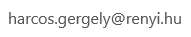﻿ BSM

## Complex Functions

• Instructor:
Gergely Harcos
Contact:• Prerequisites: Familiarity with Euclidean geometry, linear algebra over real numbers, and the arithmetic of complex numbers. Basic real analysis, including the concepts of the Riemann integral, pointwise and uniform limits, partial derivatives, and differentiability in several variables. Knowledge of measure theory and topology is not needed.
• Text: Elias M. Stein & Rami Shakarchi: Complex Analysis, Princeton University Press, 2003 ( availabe as an ebook, too)

Course description: This is an introductory course in complex analysis. We will cover the basic theorems of this beautiful subject, with detailed proofs and some illustrating examples.

Topics:

• Basics: Complex plane (arithmetic, geometry, topology), holomorphic functions, power series, integration along curves.
• Cauchy's theorems: Antiderivatives, Goursat's lemma, free homotopy, simply connected regions, Cauchy's formula.
• Applications of Cauchy's theorems: Liouville's theorem, fundamental theorem of algebra, uniqueness principle, Morera's theorem, uniform limits.
• Laurent series: Classification of isolated singularities, theorems of Riemann and Casorati-Weierstrass, meromorphic functions.
• Residue theorem and its applications: The argument principle, Rouché's theorem, Bernstein's theorem, open mapping theorem, maximum modulus principle.